# Credit Card Fraud detection using Machine Learning in Python

In this tutorial, we will learn how to make a credit card fraud detection using machine learning in Python.

In the e-commerce world, online business, cashless transactions and other informative data increase day by day. In this situation possibility of fraud also increase. Fraud can happen in many different ways. In the e-commerce transaction chance of online fraud by hacking the id and password or many other ways the fraud cases increase. This type of fraud happens in banking transactions, in government sites for information or other business transactions related fraud cases happens.

## Fraud detection by the use of various techniques: Machine Learning

The fraud detection use to know about the fraud and to take necessary action to prevent transaction fraud. There are various techniques use for fraud detection at various places for example banking transactions, Informative data, etc.  Here we discuss mainly for Machine learning and artificial intelligence use for fraud detection. Repeated method and pattern recognition used for fraud detection by making an algorithm to detect patterns.  There are various Artificial Intelligence techniques for fraud detection with the help of Data mining, Neural network, pattern recognition, Machine learning. Here, we test some techniques and their performing for credit card fraud detection.

## Building Credit card fraud detection in Python

Here, we build credit card fraud detection in five steps.

Step-1 Implementing libraries

```import pandas as pd
import numpy as np
import matplotlib.pyplot as plt
import seaborn as sns
from sklearn.model_selection import train_test_split
from sklearn.linear_model import LogisticRegression
from sklearn.metrics import confusion_matrix,accuracy_score,f1_score```

```data=pd.read_csv('creditcard.csv')

Output: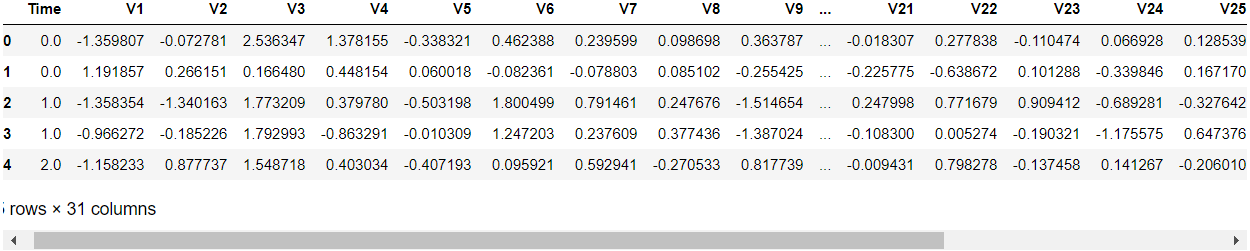Step-3 Analyze the data.

`data.describe()`

Output: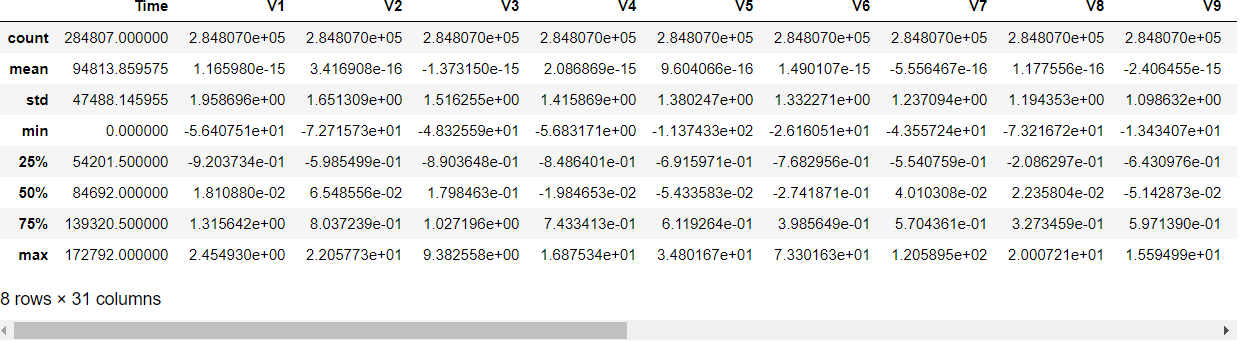Counting fraud and normal transactions. Class value 0 for normal and class value 1 for fraud.

`sns.countplot(x='Class',data=data)`

Output: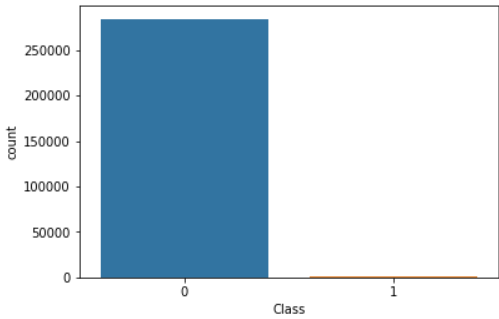Step-4 Developing a fraud detection model

Splitting the data in training and testing data.

```X=data.drop(['Class'],axis=1)
y=data['Class']
X_train,X_test,y_train,y_test=train_test_split(X,y,test_size=0.3,random_state=42)```

Initialize logistic regression and fit data into it.

```model=LogisticRegression()
model.fit(X_train,y_train)```

Predicting the value for test data.

`y_pred=model.predict(X_test)`

Step-5 Evaluating the model

Confusion metrics of model.

`confusion_matrix(y_test,y_pred)`

Output: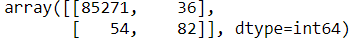F1 score of the model.

`f1_score(y_test,y_pred)`

Output: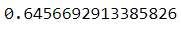Accuracy of the model.

`accuracy_score(y_test,y_pred)`

Output:

## Dataset for credit card fraud detection

Dataset has one csv file having 31 columns. It contains v1,v2, …,v28 are the principal component obtained using PCA. The class has a 0 or 1 value. 0 for a normal transaction and 1 for fraud transaction.

Dataset is available on Kaggle.